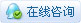260浏览

# 四旋翼飞行器控制原理与设计

### 一、相关理论知识

#### 1.坐标系与欧拉角#### 2.旋转矩阵3.四元数及其与欧拉角的关系①重力在机体坐标系下的表示：②四元数与欧拉角的关系：### 二、控制模型建立

#### 1.四旋翼动力学模型

①欧拉角表示为，②四元数表示为，（上面两个式子实际上是四元数运动学微分方程，后面姿态解算时会用到）#### 2.简化为控制模型

①先看高度通道（z方向）的化简，（俯仰、滚转都很小时）

②再看姿态通道的化简，（角度小，速度小时）

#### 3.四旋翼控制分配模型,其中是电机转速平方与拉力之间的关系系数，是电机转速平方与反扭力之间的关系系数，d是机臂长度。

### 三、控制算法及实现#### 1.姿态解算

（1）初始化四元数

``````static float q0 = 1.0f;
static float q1 = 0.0f;
static float q2 = 0.0f;
static float q3 = 0.0f;``````

（2）加速度计低通滤波，去除一部分高频噪声，获取加速度计、陀螺仪值

（3）加速度计测量值规范化

``````normalise = invSqrt(acc.x * acc.x + acc.y * acc.y + acc.z * acc.z);
acc.x *= normalise;
acc.y *= normalise;
acc.z *= normalise;``````

（4）提取四元数的等效余弦矩阵中的重力分量

``````vecxZ = 2 * (q1 * q3 - q0 * q2);
vecyZ = 2 * (q0 * q1 + q2 * q3);
veczZ = q0s - q1s - q2s + q3s;``````

（5）向量叉积得出姿态误差

``````exInt += Ki * ex * dt ;
eyInt += Ki * ey * dt ;
ezInt += Ki * ez * dt ;``````

（6）PI修正陀螺仪

``````exInt += Ki * ex * dt ;
eyInt += Ki * ey * dt ;
ezInt += Ki * ez * dt ;
gyro.x += Kp * ex + exInt;
gyro.y += Kp * ey + eyInt;
gyro.z += Kp * ez + ezInt;``````

（7）更新四元数，并归一化

``````q0 += (-q1 * gyro.x - q2 * gyro.y - q3 * gyro.z) * halfT;
q1 += (q0 * gyro.x + q2 * gyro.z - q3 * gyro.y) * halfT;
q2 += (q0 * gyro.y - q1 * gyro.z + q3 * gyro.x) * halfT;
q3 += (q0 * gyro.z + q1 * gyro.y - q2 * gyro.x) * halfT;

normalise = invSqrt(q0 * q0 + q1 * q1 + q2 * q2 + q3 * q3);
q0 *= normalise;
q1 *= normalise;
q2 *= normalise;
q3 *= normalise;``````（8）解算欧拉角

``````
state->attitude.roll = atan2f(vecyZ, veczZ) * RAD2DEG;
state->attitude.yaw = atan2f(2 * (q1 * q2 + q0 * q3),q0s + q1s - q2s - q3s) * RAD2DEG;``````

2.串级PID控制

PID控制器的设计是基于控制模型的，在前面说过，我们设计的PID控制如下，

①姿态环``````void attitudeRatePID(Axis3f *actualRate,attitude_t *desiredRate,control_t *output)
{
output->roll = pidOutLimit(pidUpdate(&pidRateRoll, desiredRate->roll - actualRate->x));
output->pitch = pidOutLimit(pidUpdate(&pidRatePitch, desiredRate->pitch - actualRate->y));
output->yaw = pidOutLimit(pidUpdate(&pidRateYaw, desiredRate->yaw - actualRate->z));
}

void attitudeAnglePID(attitude_t *actualAngle,attitude_t *desiredAngle,attitude_t *outDesiredRate)
{
outDesiredRate->roll = pidUpdate(&pidAngleRoll, desiredAngle->roll - actualAngle->roll);
outDesiredRate->pitch = pidUpdate(&pidAnglePitch, desiredAngle->pitch - actualAngle->pitch);

float yawError = desiredAngle->yaw - actualAngle->yaw ;
if (yawError > 180.0f)
yawError -= 360.0f;
else if (yawError < -180.0)
yawError += 360.0f;
outDesiredRate->yaw = pidUpdate(&pidAngleYaw, yawError);
}``````

②高度环``````void altholdPID(float* thrust, const state_t *state, const setpoint_t *setpoint)
{
float newThrust = 0.0;

newThrust = THRUST_SCALE * runPidZ(&posPid.pidVZ, state->position.z, setpoint, POS_UPDATE_DT);

if(getCommanderKeyFlight())
detecWeight(*thrust, newThrust, state->velocity.z);

*thrust = newThrust + posPid.thrustBase;

if (*thrust > 60000)
{
*thrust = 60000;
}
}``````
``````static float runPidZ(pidAxis_t *axis, float input, const setpoint_t *setpoint, float dt)
{
float out = 0.f;
if (axis->preMode == false && setpoint->isAltHold == true)
{
positionResetAllPID();
axis->setpoint = input + START_HIRHT;
posPid.thrustBase = limitThrustBase(configParam.thrustBase);
}
axis->preMode = setpoint->isAltHold;

if(setpoint->isAltHold == true)
{
axis->setpoint += setpoint->velocity.z * dt;
out = pidUpdate(&axis->pid, axis->setpoint - input);
}
return out;
}``````

pid更新的代码，

``````float pidUpdate(PidObject* pid, const float error)
{
float output;

pid->error = error;

pid->integ += pid->error * pid->dt;
if (pid->integ > pid->iLimit)
{
pid->integ = pid->iLimit;
}
else if (pid->integ < pid->iLimitLow)
{
pid->integ = pid->iLimitLow;
}

pid->deriv = (pid->error - pid->prevError) / pid->dt;

pid->outP = pid->kp * pid->error;
pid->outI = pid->ki * pid->integ;
pid->outD = pid->kd * pid->deriv;

output = pid->outP + pid->outI + pid->outD;

pid->prevError = pid->error;

return output;
}``````

pid算法虽然都是一样的，但是基于pid控制器，不同飞控实现的方式也不太一样，这里不过多解读minifly的飞控了，后面好好学学px4的源码。

#### 3.控制分配

``````void powerControl(control_t *control)
{
s16 r = control->roll / 2.0f;
s16 p = control->pitch / 2.0f;

motorPWM.m1 = limitThrust(control->thrust - r - p + control->yaw);
motorPWM.m2 = limitThrust(control->thrust - r + p - control->yaw);
motorPWM.m3 = limitThrust(control->thrust + r + p + control->yaw);
motorPWM.m4 = limitThrust(control->thrust + r - p - control->yaw);
}``````

 膜拜大佬

 您需要登录后才可以回帖 登录 | 立即注册 本版积分规则 回帖并转播 回帖后跳转到最后一页

[[wsData.name]]

#### 硬件清单

• [[d.name]]#### 楼主的其它帖子